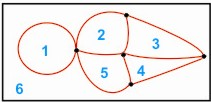HOME MATH DICTIONARY DOWNLOAD FEEDBACK DISCLAIMER
 Question: What is Region ? Answer: A Circle drawn on a page divides it into two regions, that part of the surface inside the circle and that part outside. When a network is drawn on a piece of paper it will divide it into a number of regions each bounded by arcs. In the example there are 6 regions, 5 inside the network and one region outside.When a graph is drawn for an equation such as x + y = 6, the line representing it divides the plane into two regions which corresponds to the inequalities x + y > 6 and x + y < 6 . Equations such as y = x2 divide the plane in a similar way but this time the boundary line is a parabola.